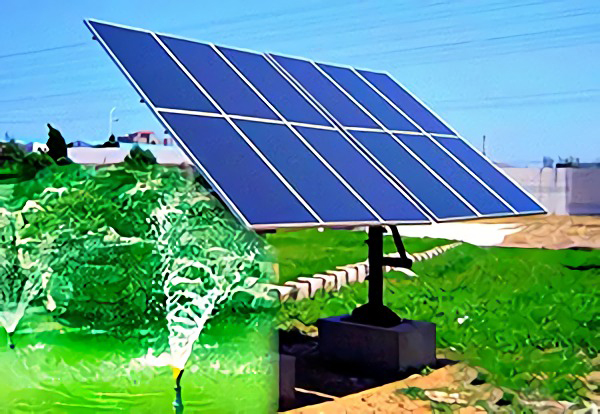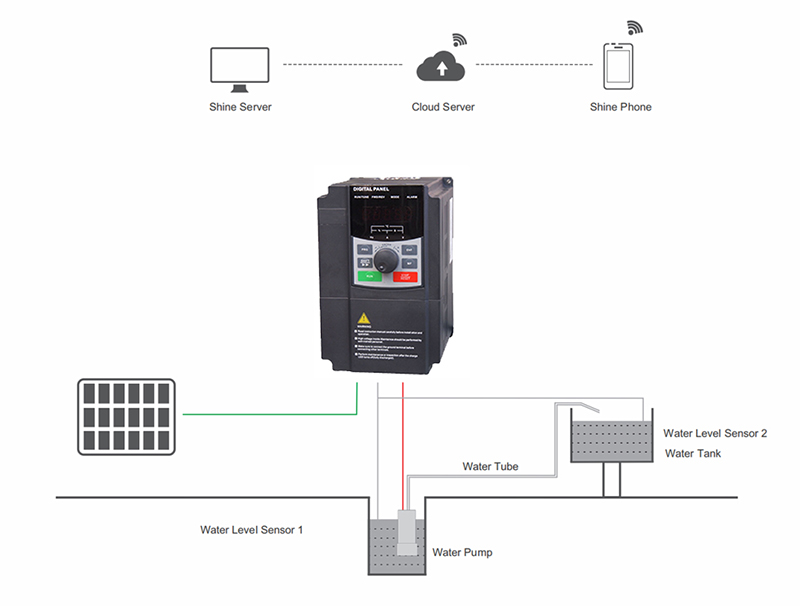# Solar Pump Inverter Functions

Solar pump inverter is used to control and regulate the operation of solar water pump system (PV pumping system). It can convert the DC from the solar array into AC to drive the water pump. In addition, it can adjust the output frequency in real time according to the sunlight intensity so as to achieve maximum power point tracking (MPPT).

Solar pump inverter is one of the various types of power inverters. Its function is similar with the function of common on grid inverter. The two inverters are both used to convert solar DC into AC. But the solar pump inverter is greatly improved. Thus, it can greatly save the system cost and can expand the solar energy application range.

Solar pump inverter is an off grid inverter. It does not rely on the power grid and can drive the load independently. However, the routine off grid inverter can work only when it is equipped with battery. But the lead-acid battery price is very high, accounting 30% of the total system cost. Besides, its service life is as short as 3-5 years, which will affect the system investment income.

The solar pump inverter does not need battery and can work as long as there is sunlight. What we need do is to construct a water tower at the high place and we can take the water from this water tower any time we need it. The inverter itself will be installed with water level control switch, which is very convenient. Its role is similar with the role of the battery in off-grid system. But the water tower cost is much lower than battery cost.

In the off-grid system, the most tough load is the motor because it needs high power to start the motor. The start power of the routine motor is about 3 times of the rated power. At the same time, as the water pump motor needs to pump water to the high place, its start power is about 5 times of the rated power. If the water pump motor is driven by routine off grid inverter, the start power needs to be amplified for 5 times. For example, if the water pump motor’s power is 2kW, it can only be driven by the off grid inverter with 10kW power. In addition, even in the normal operation process, the DC terminal input power shall be over 2kW to ensure the continuous operation of the motor. This will increase the system cost.To solve this problem, the special algorithm is added on the solar water pump inverter and the power only needs to be increased by 20%. For instance, the 4kW water motor can be started by a 5.5kW solar pump inverter. To maintain the operation of the water pump, the solar input power does not need to reach 4kW and only 1kW is enough.

Why the function of solar water pump inverter is so amazing? The explanation shall be started from the AC work principle. The AC includes three elements which are voltage, current and frequency. Normally, the frequency is 50HZ. When the motor is started, it rotates 50 times per second. The power changes with the change of the current and voltage. Thus, the voltage and current are used to calculate the power. But the situation is different for the motor and its power is affected by the frequency. Its rated power is the power calculated under the 50Hz frequency. When the frequency decreases, the power will decrease accordingly. The rated power of the motor =rated torque*rated rotation times. As long as the rated torque is not changed, the motor can work normally. Thus, when the frequency and voltage decreases, the rated power and rotation speed (frequency) will decrease proportionally.The frequency transforming function is added on the solar pump inverter, which can change the AC output frequency. Thus, when starting the motor, the frequency can be decreased so as to decrease the power. After the motor is started, the frequency can be increased accordingly to increase the rotation speed. In addition, the frequency also changes with the change of the sunlight. This is why the 4kW water pump can be driven by only 1kW solar energy input.

Since the solar pump inverter has so excellent function, can we expand its application range? Unfortunately, it can not be expanded. As the output voltage, current, phase and frequency of solar pump inverter change with the changing of the sunlight, it is not applicable in my situations. The main reasons are as following:

1. Due to the technology limitation, solar pump inverters can not be used in parallel. One inverter can only be connected with one motor. Thus, the maximum power is limited. By far, the solar pump inverter that can support energy storage function is not created yet. In the areas with lower temperature in winter, the external water tower with freeze and the solar water pumping system will be limited. In addition, the solar pump inverter can not be connected with the power grid because the power grid requires synchronous frequency and voltage.
2. The solar pump inverter can only carry limited load. By far, it can only carry the water pump motor but can not carry the load that requires stable power and specific voltage and frequency. For instance, the water inverter can not be used to drive electric lamps, refrigerators, computers, washing machines, even the variable frequency air conditioners. It can only drive the pure resistive-load water heater.